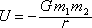This is the universal-law-of-gravitation expression for gravitational potential energy. The gravitational potential energy U (written UG if it needs to be distinguished from other kinds of potential energy) for a pair of objects is the negative of the universal gravitational constant G times the product of the masses of the objects, all divided by the separation r of the centers of masses of the objects.

Note: The expression is exact for point particles and for cases in which the objects do not overlap and both of the objects are spherically symmetric. For other cases, it is a good approximation as long as the length of the longer object is small compared to the separation of the centers of mass.

Pitfall Avoidance Notes: The r in the denominator is not squared. Also, the r is the center-to-center distance, not the surface-to-surface distance.